# NLP的文本分析与特征工程

### 摘要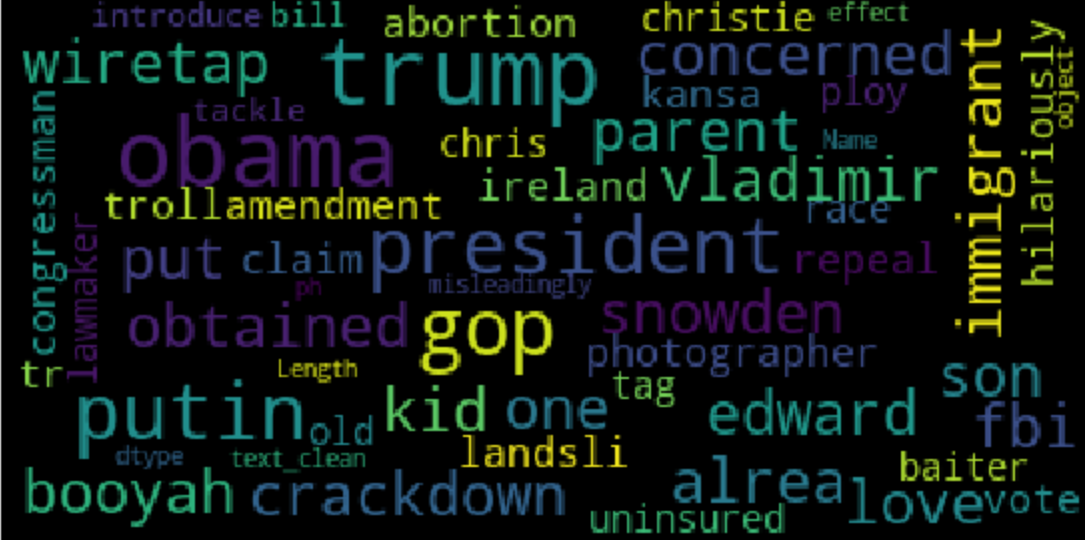NLP常用于文本数据的分类。文本分类是根据文本数据的内容对其进行分类的问题。文本分类最重要的部分是特征工程：从原始文本数据为机器学习模型创建特征的过程。

https://github.com/mdipietro09/DataScience_ArtificialIntelligence_Utils/blob/master/deep_learning_natural_language_processing/text_classification_example.ipynb

https://www.kaggle.com/rmisra/news-category-dataset

• 环境设置：导入包并读取数据。

• 语言检测：了解哪些自然语言数据在其中。

• 文本预处理：文本清理和转换。

• 长度分析：用不同的指标来衡量。

• 情绪分析：判断一篇文章是正面的还是负面的。

• 命名实体识别：带有预定义类别（如人名、组织、位置）的标识文本。

• 词频：找出最重要的n个字母。

• 词向量：把一个字转换成向量。

• 主题模型：从语料库中提取主题。

### 环境设置

## 数据
import pandas as pd
import collections
import json
## 绘图
import matplotlib.pyplot as plt
import seaborn as sns
import wordcloud
## 文本处理
import re
import nltk
## 语言检测
import langdetect
## 情感分析
from textblob import TextBlob
## 命名实体识别
import spacy
## 词频
from sklearn import feature_extraction, manifold
## word embedding
## 主题模型
import gensim


lst_dics = []
with open('data.json', mode='r', errors='ignore') as json_file:
for dic in json_file:
## 打印第一个
lst_dics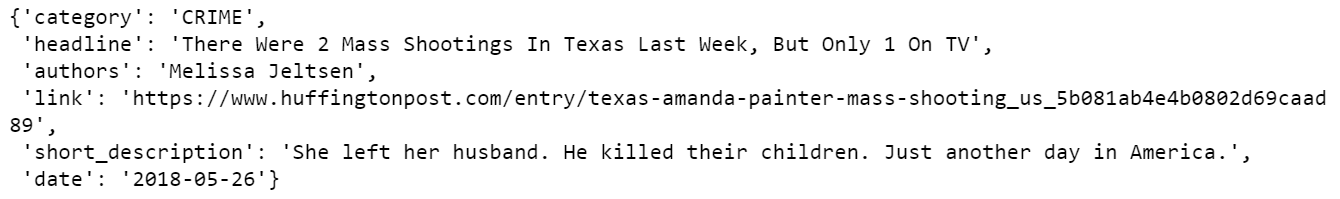## 创建dtf
dtf = pd.DataFrame(lst_dics)
## 筛选类别
## 重命名列
## 打印5个随机行
dtf.sample(5)x = "y"
fig, ax = plt.subplots()
fig.suptitle(x, fontsize=12)
dtf[x].reset_index().groupby(x).count().sort_values(by=
"index").plot(kind="barh", legend=False,
ax=ax).grid(axis='x')
plt.show()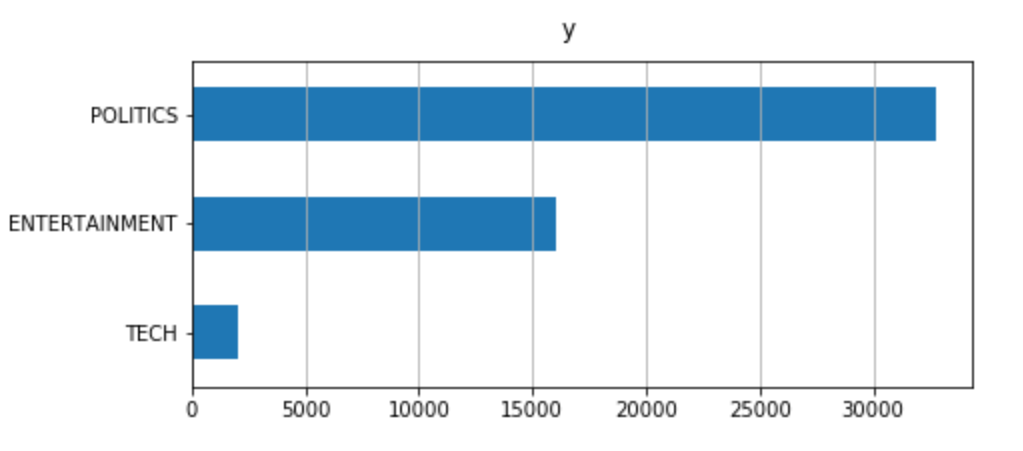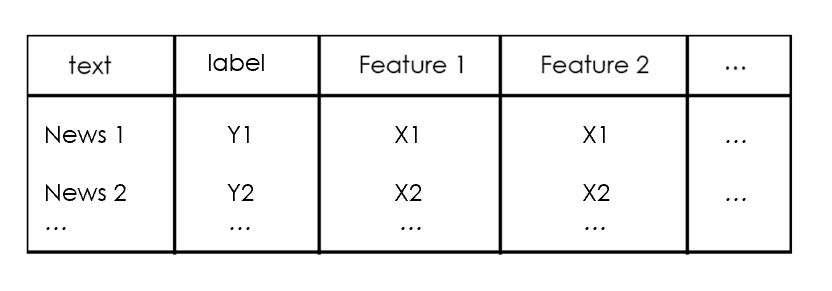### 语言检测

txt = dtf["text"].iloc
print(txt, " --> ", langdetect.detect(txt))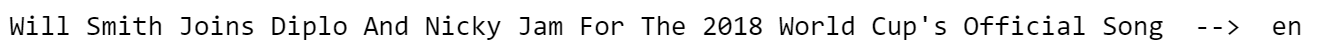dtf['lang'] = dtf["text"].apply(lambda x: langdetect.detect(x) if
x.strip() != "" else "")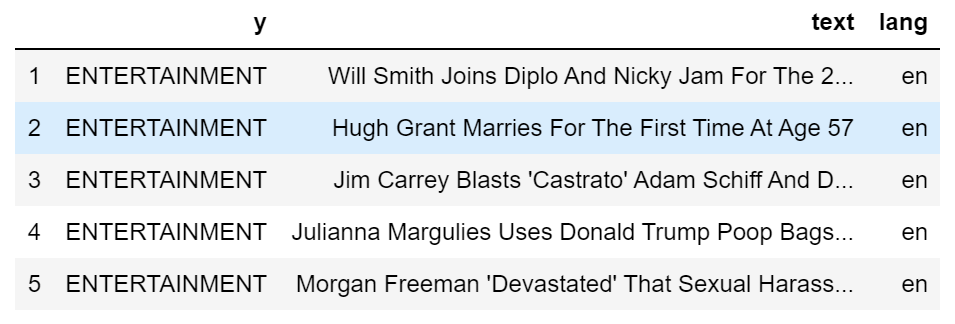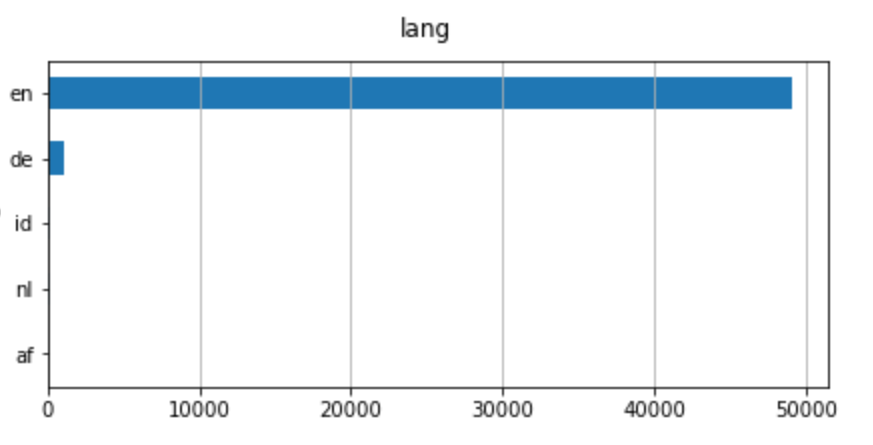dtf = dtf[dtf["lang"]=="en"]


### 文本预处理

print("--- original ---")
print(txt)
print("--- cleaning ---")
txt = re.sub(r'[^\w\s]', '', str(txt).lower().strip())
print(txt)
print("--- tokenization ---")
txt = txt.split()
print(txt)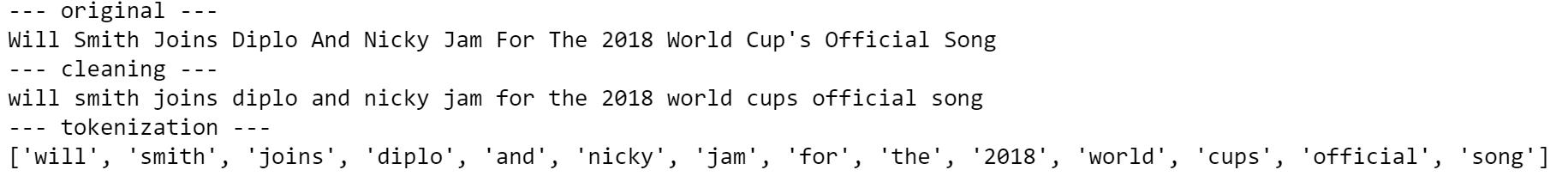lst_stopwords = nltk.corpus.stopwords.words("english")
lst_stopwords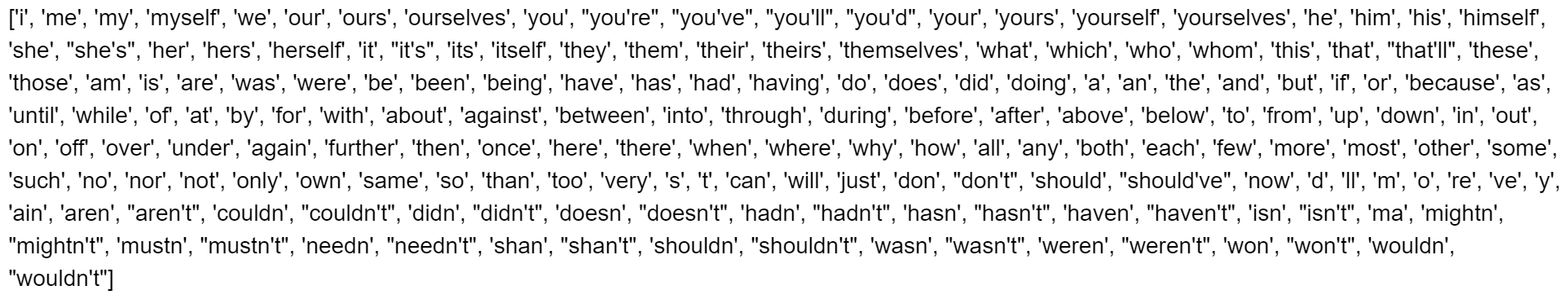print("--- remove stopwords ---")
txt = [word for word in txt if word not in lst_stopwords]
print(txt)


print("--- stemming ---")
ps = nltk.stem.porter.PorterStemmer()
print([ps.stem(word) for word in txt])
print("--- lemmatisation ---")
lem = nltk.stem.wordnet.WordNetLemmatizer()
print([lem.lemmatize(word) for word in txt])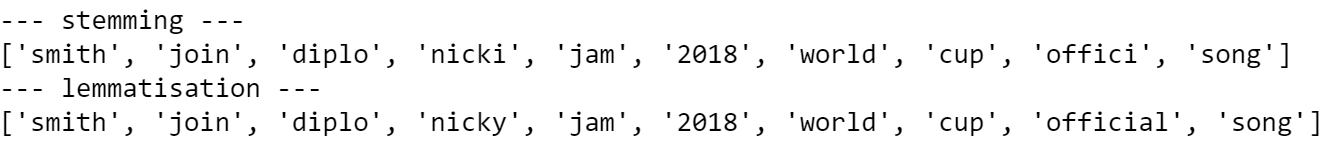'''

:parameter
:param text: string - 包含文本的列的名称
:param lst_stopwords: list - 要删除的停用词列表
:param flg_stemm: bool - 是否应用词干
:param flg_lemm: bool - 是否应用引理化
:return
cleaned text
'''
def utils_preprocess_text(text, flg_stemm=False, flg_lemm=True, lst_stopwords=None):
## 清洗（转换为小写并删除标点和字符，然后删除）
text = re.sub(r'[^\w\s]', '', str(text).lower().strip())

## 标识化（从字符串转换为列表）
lst_text = text.split()
## 删除停用词
if lst_stopwords is not None:
lst_text = [word for word in lst_text if word not in
lst_stopwords]

## 词干化
if flg_stemm == True:
ps = nltk.stem.porter.PorterStemmer()
lst_text = [ps.stem(word) for word in lst_text]

## 引理化
if flg_lemm == True:
lem = nltk.stem.wordnet.WordNetLemmatizer()
lst_text = [lem.lemmatize(word) for word in lst_text]

## 从列表返回到字符串
text = " ".join(lst_text)
return text


dtf["text_clean"] = dtf["text"].apply(lambda x: utils_preprocess_text(x, flg_stemm=False, flg_lemm=True, lst_stopwords))


dtf.head()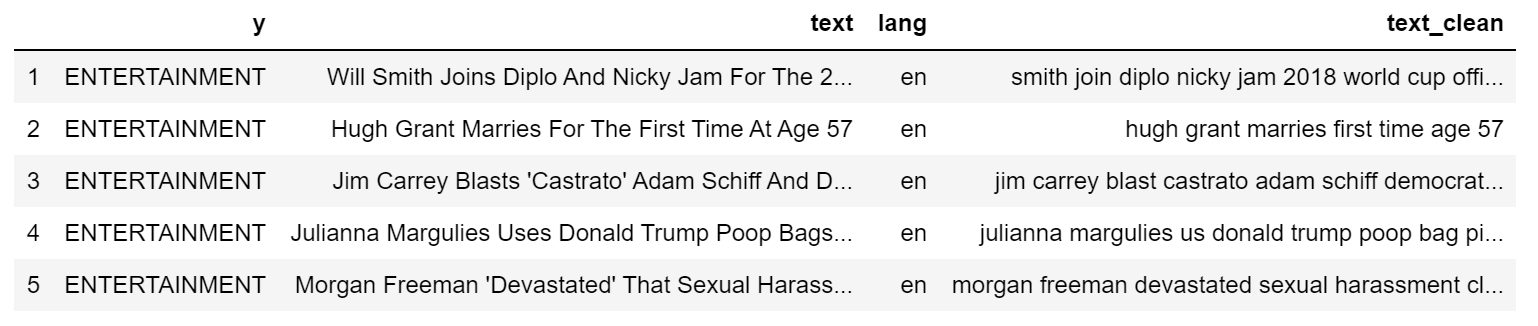print(dtf["text"].iloc, " --> ", dtf["text_clean"].iloc)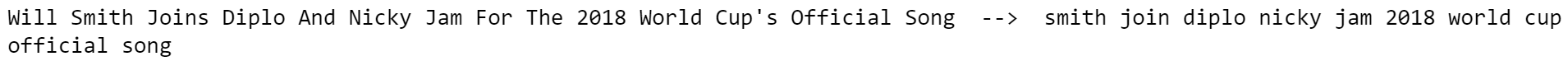### 长度分析

• 字数：统计文本中的标识数（用空格分隔）
• 字符数：将每个标识的字符数相加
• 句子计数：计算句子的数量（用句点分隔）
• 平均字长：字长之和除以字数（字数/字数）
• 平均句子长度：句子长度之和除以句子数（字数/句子数）
dtf['word_count'] = dtf["text"].apply(lambda x: len(str(x).split(" ")))
dtf['char_count'] = dtf["text"].apply(lambda x: sum(len(word) for word in str(x).split(" ")))
dtf['sentence_count'] = dtf["text"].apply(lambda x: len(str(x).split(".")))
dtf['avg_word_length'] = dtf['char_count'] / dtf['word_count']
dtf['avg_sentence_lenght'] = dtf['word_count'] / dtf['sentence_count']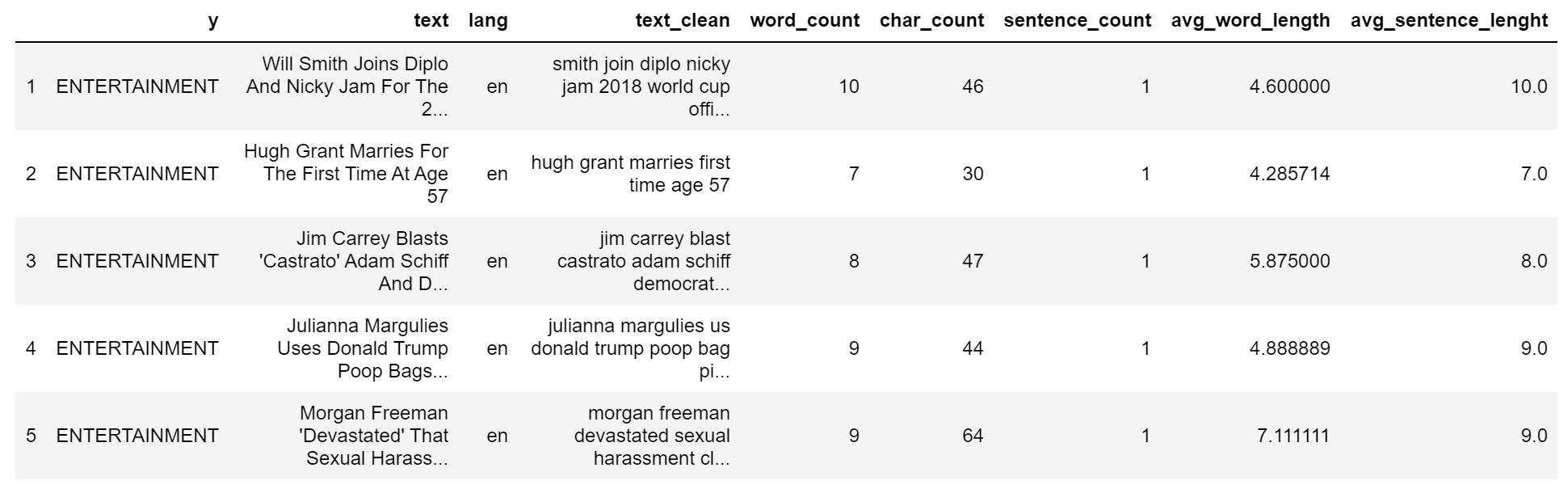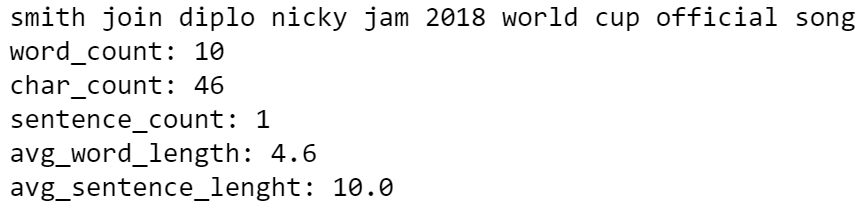x, y = "char_count", "y"
fig, ax = plt.subplots(nrows=1, ncols=2)
fig.suptitle(x, fontsize=12)
for i in dtf[y].unique():
sns.distplot(dtf[dtf[y]==i][x], hist=True, kde=False,
bins=10, hist_kws={"alpha":0.8},
axlabel="histogram", ax=ax)
sns.distplot(dtf[dtf[y]==i][x], hist=False, kde=True,
ax=ax)
ax.grid(True)
ax.legend(dtf[y].unique())
ax.grid(True)
plt.show()


### 情感分析

• Textblob建立在NLTK的基础上，是最流行的一种，它可以给单词赋予极性，并作为一个平均值来估计整个文本的情绪。
• 另一方面，Vader（Valence-aware dictionary and mootion reasoner）是一个基于规则的模型，尤其适用于社交媒体数据。

dtf["sentiment"] = dtf[column].apply(lambda x:
TextBlob(x).sentiment.polarity)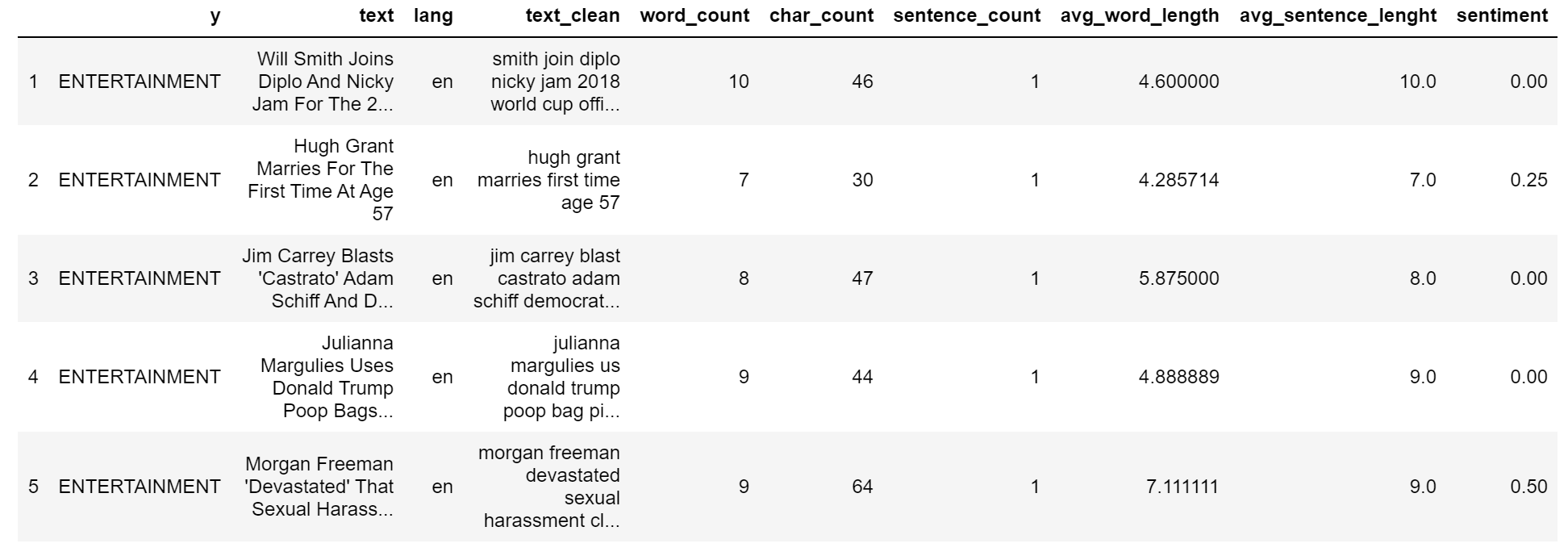print(dtf["text"].iloc, " --> ", dtf["sentiment"].iloc)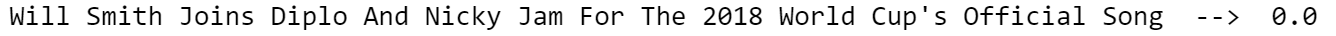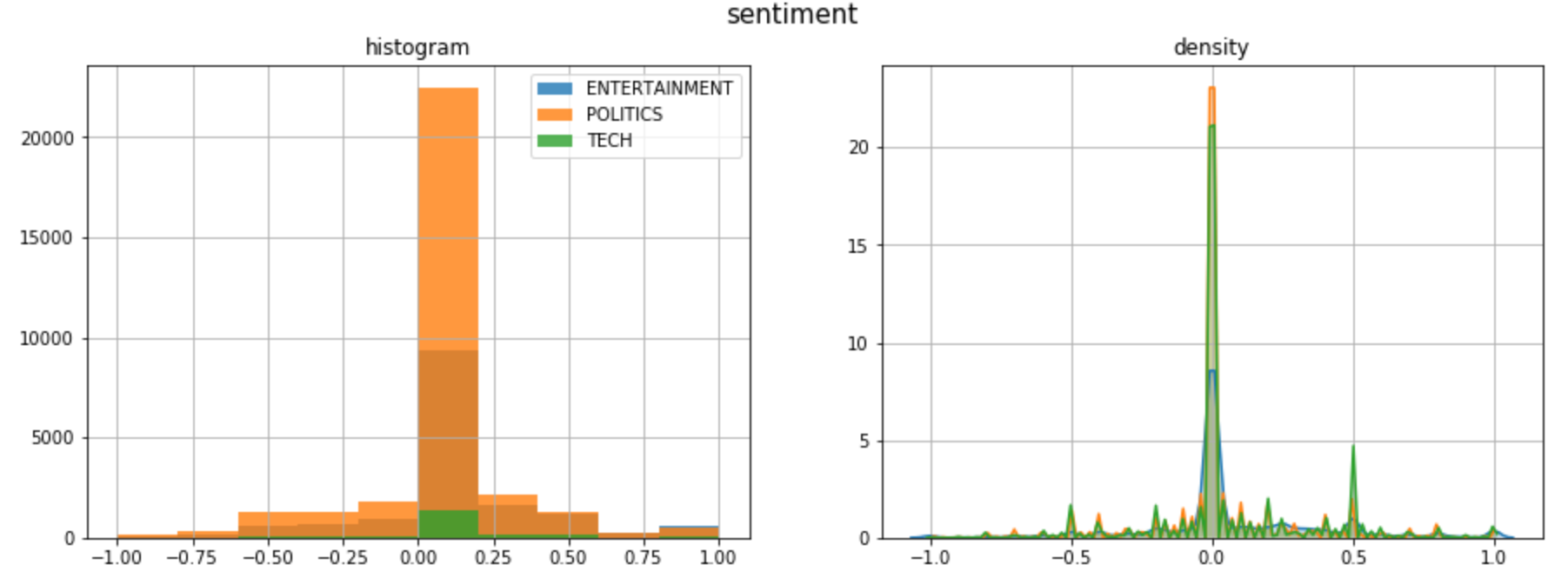### 命名实体识别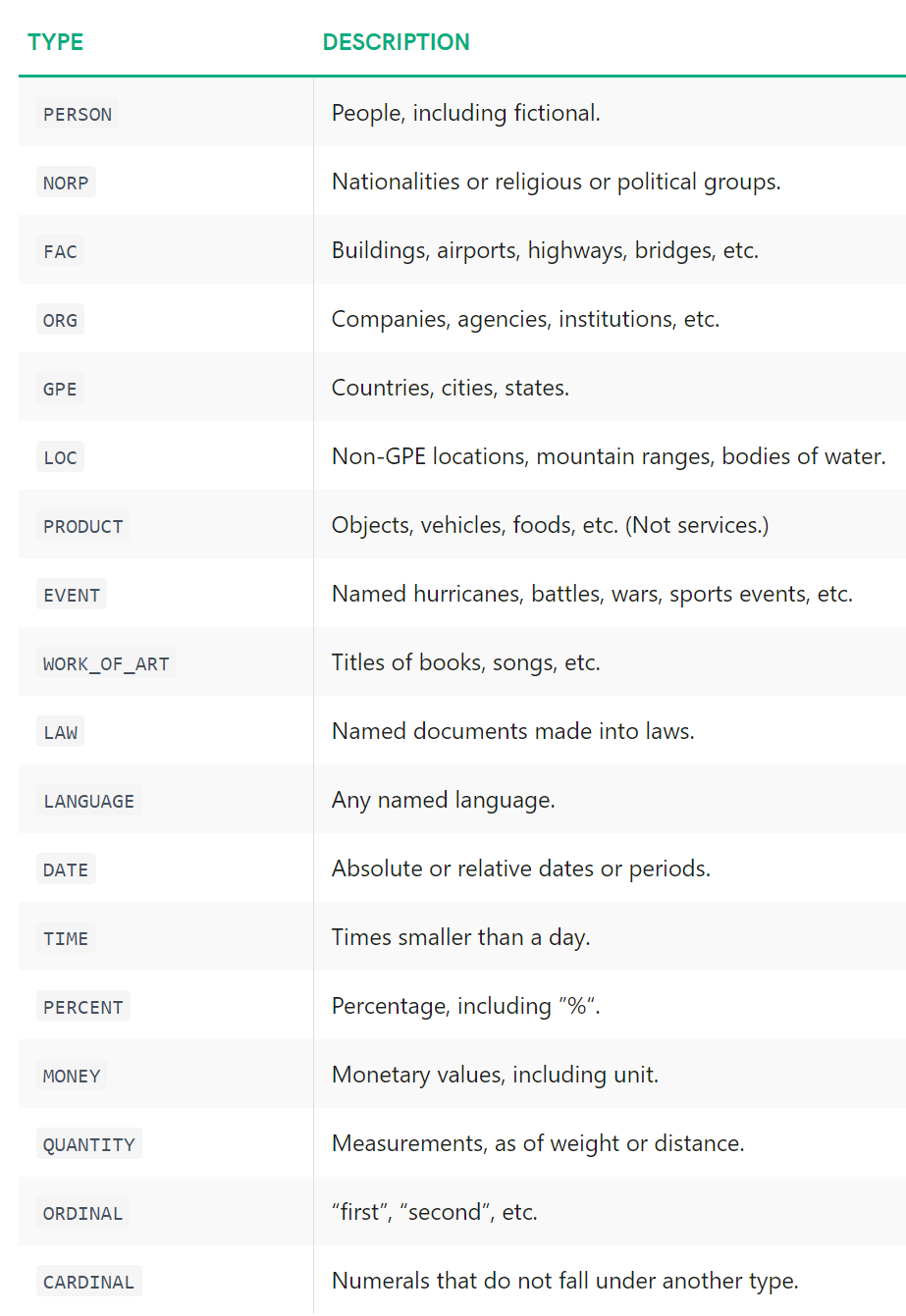## 调用
## 打标签
txt = dtf["text"].iloc
doc = ner(txt)
## 展示结果
spacy.displacy.render(doc, style="ent")• 对数据集中的每个文本观察运行NER模型，就像我在前面的示例中所做的那样。

• 对于每个新闻标题，我将把所有被认可的实体以及同一实体出现在文本中的次数放入一个新的列（称为“tags”）。

在这个例子中：

{ (‘Will Smith’, ‘PERSON’):1,
(‘Diplo’, ‘PERSON’):1,
(‘Nicky Jam’, ‘PERSON’):1,
(“The 2018 World Cup’s”, ‘EVENT’):1 }

• 然后，我将为每个标识类别（Person、Org、Event，…）创建一个新列，并计算每个标识类别找到的实体数。在上面的例子中，特征将是

tags_PERSON = 3

tags_EVENT = 1

## 标识文本并将标识导出到列表中
dtf["tags"] = dtf["text"].apply(lambda x: [(tag.text, tag.label_)
for tag in ner(x).ents] )
## utils函数计算列表元素
def utils_lst_count(lst):
dic_counter = collections.Counter()
for x in lst:
dic_counter[x] += 1
dic_counter = collections.OrderedDict(
sorted(dic_counter.items(),
key=lambda x: x, reverse=True))
lst_count = [ {key:value} for key,value in dic_counter.items() ]
return lst_count

## 计数
dtf["tags"] = dtf["tags"].apply(lambda x: utils_lst_count(x))

## utils函数为每个标识类别创建新列
def utils_ner_features(lst_dics_tuples, tag):
if len(lst_dics_tuples) > 0:
tag_type = []
for dic_tuples in lst_dics_tuples:
for tuple in dic_tuples:
type, n = tuple, dic_tuples[tuple]
tag_type = tag_type + [type]*n
dic_counter = collections.Counter()
for x in tag_type:
dic_counter[x] += 1
return dic_counter[tag]
else:
return 0

## 提取特征
tags_set = []
for lst in dtf["tags"].tolist():
for dic in lst:
for k in dic.keys():
tags_set.append(k)
tags_set = list(set(tags_set))
for feature in tags_set:
dtf["tags_"+feature] = dtf["tags"].apply(lambda x:
utils_ner_features(x, feature))

## 结果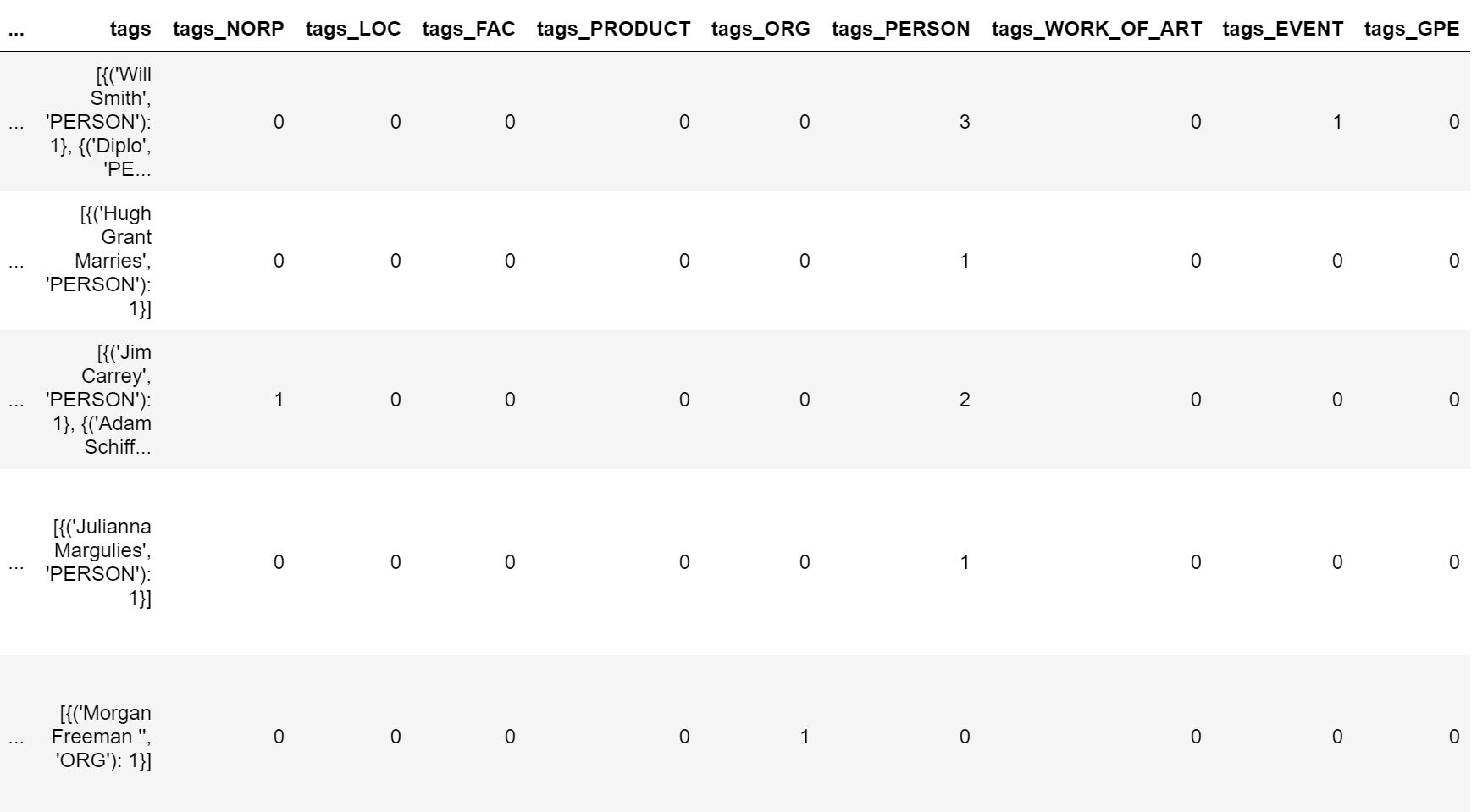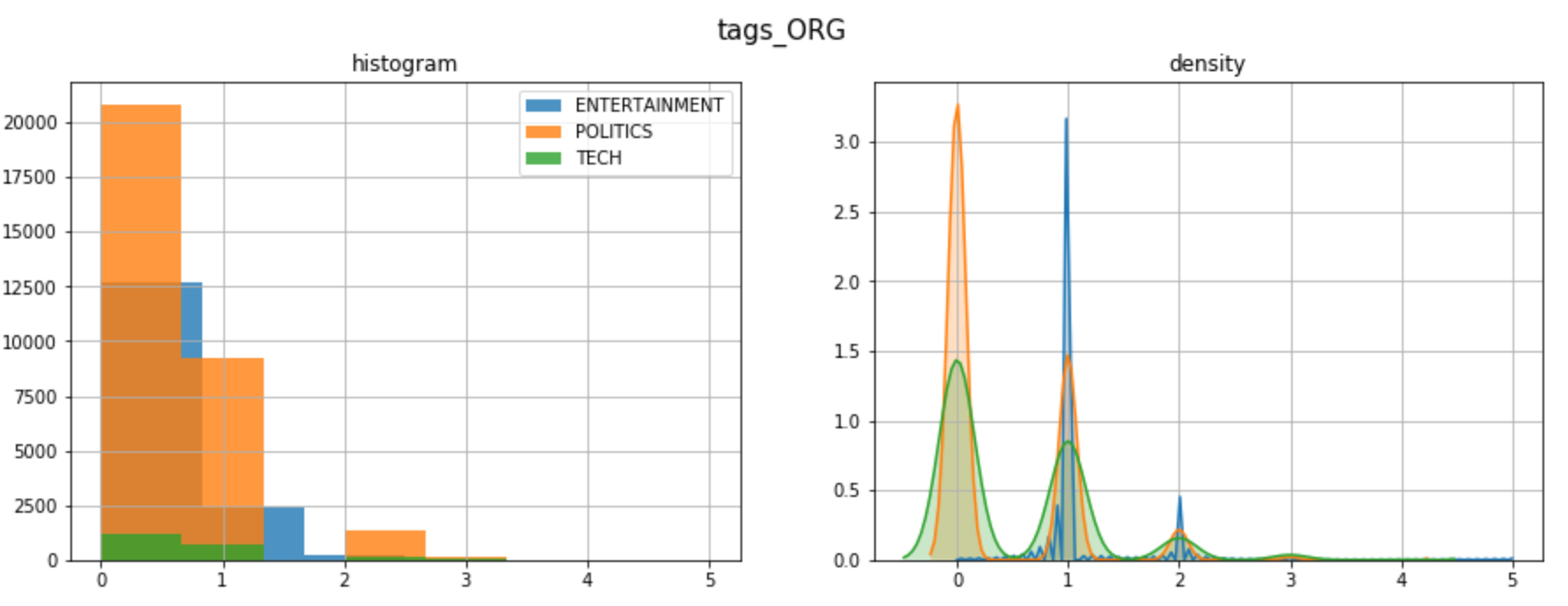y = "ENTERTAINMENT"

tags_list = dtf[dtf["y"]==y]["tags"].sum()
map_lst = list(map(lambda x: list(x.keys()), tags_list))
dtf_tags = pd.DataFrame(map_lst, columns=['tag','type'])
dtf_tags["count"] = 1
dtf_tags = dtf_tags.groupby(['type',
'tag']).count().reset_index().sort_values("count",
ascending=False)
fig, ax = plt.subplots()
fig.suptitle("Top frequent tags", fontsize=12)
sns.barplot(x="count", y="tag", hue="type",
data=dtf_tags.iloc[:top,:], dodge=False, ax=ax)
ax.grid(axis="x")
plt.show()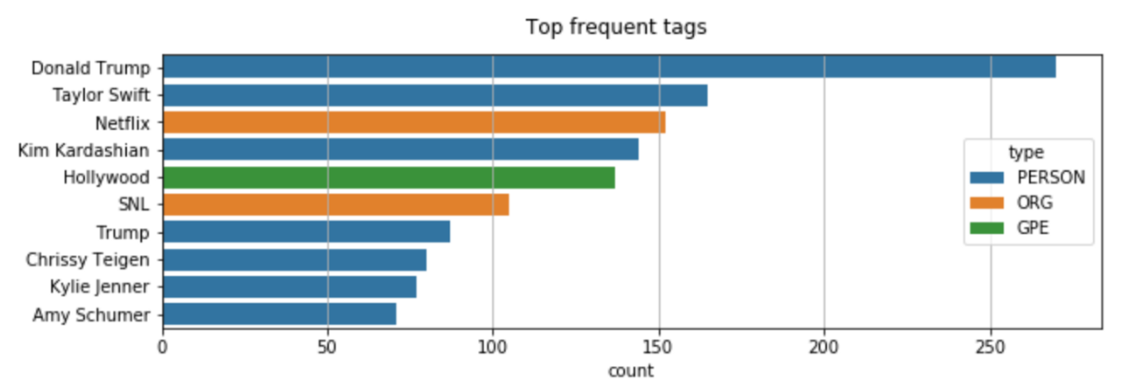## 预测
txt = dtf["text"].iloc
entities = ner(txt).ents
## 打标签
tagged_txt = txt
for tag in entities:
tagged_txt = re.sub(tag.text, "_".join(tag.text.split()),
tagged_txt)
## 结果
print(tagged_txt)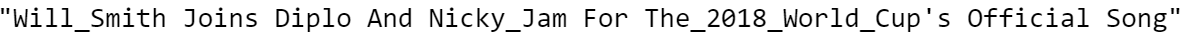### 词频

• 4个unigram： “I”, “like”, “this”, “article

y = "POLITICS"
corpus = dtf[dtf["y"]==y]["text_clean"]
lst_tokens = nltk.tokenize.word_tokenize(corpus.str.cat(sep=" "))
fig, ax = plt.subplots(nrows=1, ncols=2)
fig.suptitle("Most frequent words", fontsize=15)

## unigrams
dic_words_freq = nltk.FreqDist(lst_tokens)
dtf_uni = pd.DataFrame(dic_words_freq.most_common(),
columns=["Word","Freq"])
dtf_uni.set_index("Word").iloc[:top,:].sort_values(by="Freq").plot(
kind="barh", title="Unigrams", ax=ax,
legend=False).grid(axis='x')
ax.set(ylabel=None)

## bigrams
dic_words_freq = nltk.FreqDist(nltk.ngrams(lst_tokens, 2))
dtf_bi = pd.DataFrame(dic_words_freq.most_common(),
columns=["Word","Freq"])
dtf_bi["Word"] = dtf_bi["Word"].apply(lambda x: " ".join(
string for string in x) )
dtf_bi.set_index("Word").iloc[:top,:].sort_values(by="Freq").plot(
kind="barh", title="Bigrams", ax=ax,
legend=False).grid(axis='x')
ax.set(ylabel=None)
plt.show()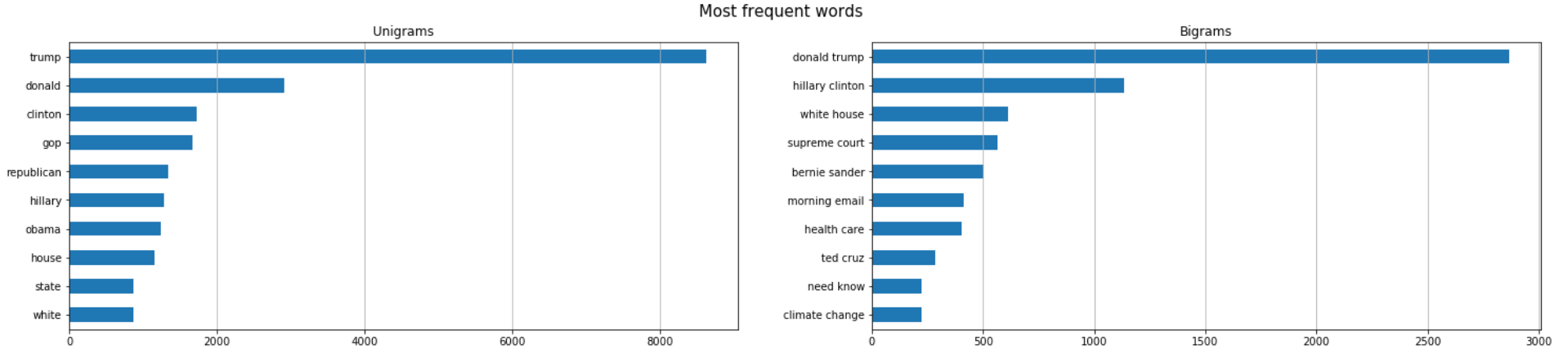CountVectorizer将文本文档集合转换为计数矩阵。我将用3个n-grams来举例：“box office”（经常出现在娱乐圈）、“republican”（经常出现在政界）、“apple”（经常出现在科技界）。

lst_words = ["box office", "republican", "apple"]
## 计数
lst_grams = [len(word.split(" ")) for word in lst_words]
vectorizer = feature_extraction.text.CountVectorizer(
vocabulary=lst_words,
ngram_range=(min(lst_grams),max(lst_grams)))
dtf_X = pd.DataFrame(vectorizer.fit_transform(dtf["text_clean"]).todense(), columns=lst_words)
## 将新特征添加为列
dtf = pd.concat([dtf, dtf_X.set_index(dtf.index)], axis=1)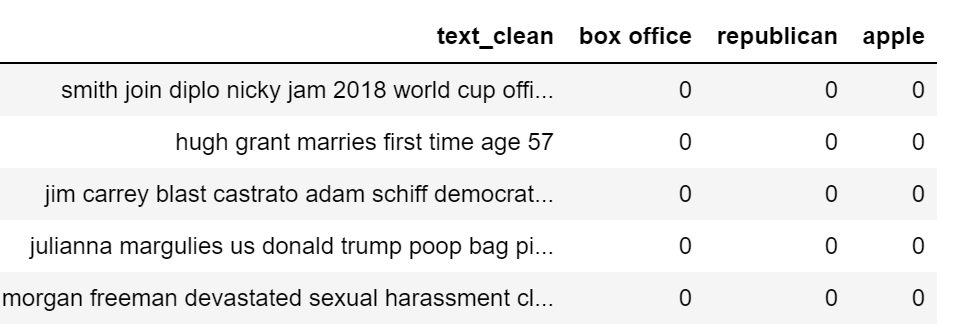wc = wordcloud.WordCloud(background_color='black', max_words=100,
max_font_size=35)
wc = wc.generate(str(corpus))
fig = plt.figure(num=1)
plt.axis('off')
plt.imshow(wc, cmap=None)
plt.show()### 词向量

GloVe是一种无监督学习算法，用于获取300个单词的向量表示。

nlp = gensim_api.load("glove-wiki-gigaword-300")


word = "love"
nlp[word]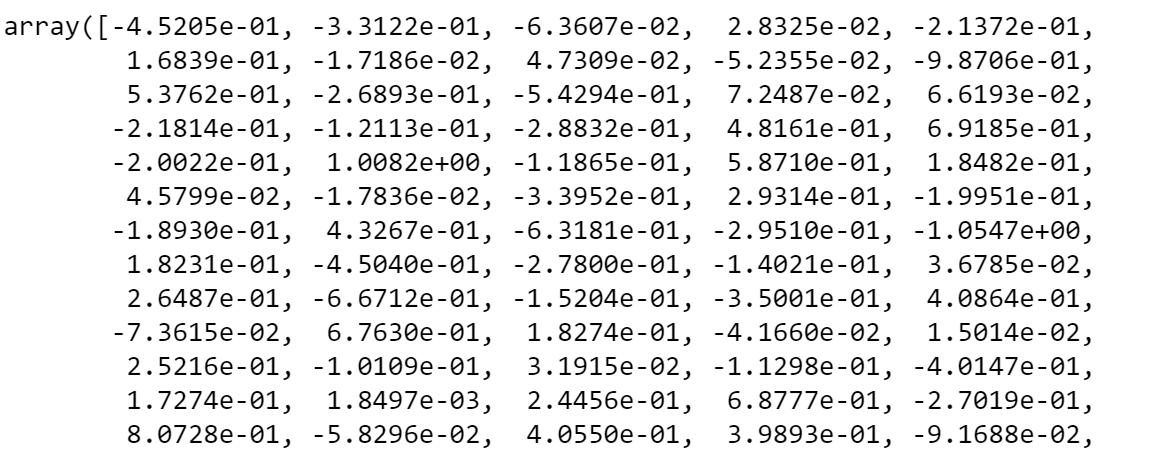nlp[word].shape


t-SNE是一种可视化高维数据的工具，它将数据点之间的相似性转换为联合概率。

## 找到最近的向量
labels, X, x, y = [], [], [], []
for t in nlp.most_similar(word, topn=20):
X.append(nlp[t])
labels.append(t)
## 降维
pca = manifold.TSNE(perplexity=40, n_components=2, init='pca')
new_values = pca.fit_transform(X)
for value in new_values:
x.append(value)
y.append(value)
## 绘图
fig = plt.figure()
for i in range(len(x)):
plt.scatter(x[i], y[i], c="black")
plt.annotate(labels[i], xy=(x[i],y[i]), xytext=(5,2),
textcoords='offset points', ha='right', va='bottom')
## 添加中心
plt.scatter(x=0, y=0, c="red")
plt.annotate(word, xy=(0,0), xytext=(5,2), textcoords='offset
points', ha='right', va='bottom')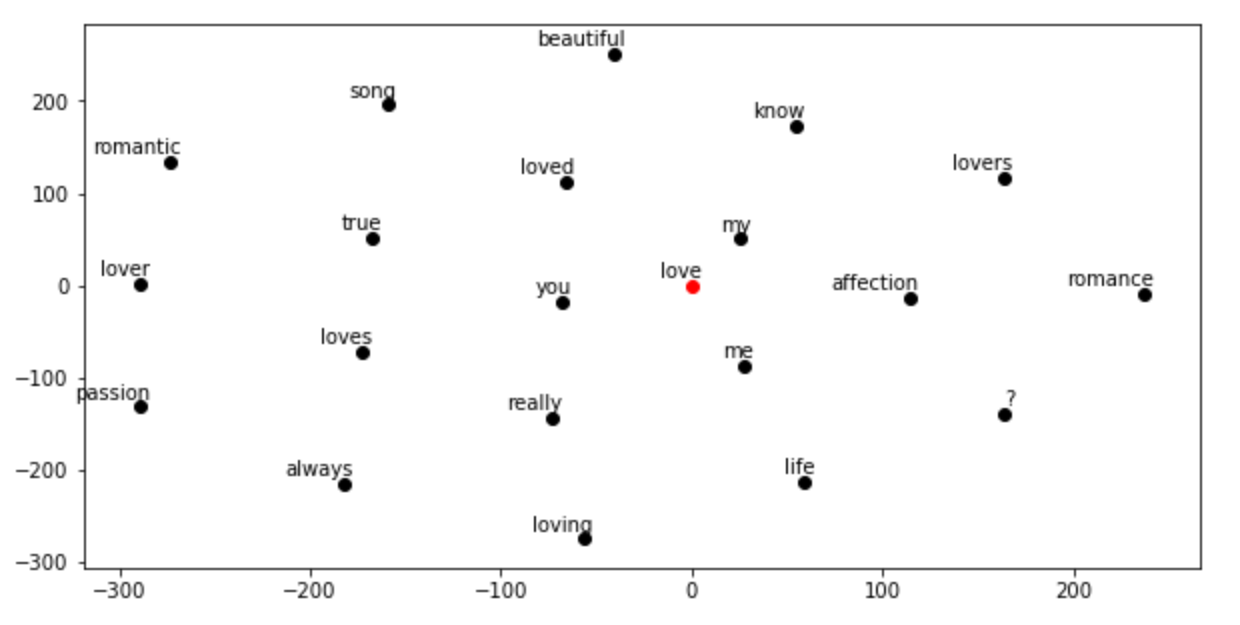### 主题模型

Genism包专门用于主题模型。主题模型是一种用于发现文档集合中出现的抽象“主题”的统计模型。

y = "TECH"
corpus = dtf[dtf["y"]==y]["text_clean"]

## 预处理语料库
lst_corpus = []
for string in corpus:
lst_words = string.split()
lst_grams = [" ".join(lst_words[i:i + 2]) for i in range(0,
len(lst_words), 2)]
lst_corpus.append(lst_grams)
## 将单词映射到id
id2word = gensim.corpora.Dictionary(lst_corpus)
## 创建词典 word:freq
dic_corpus = [id2word.doc2bow(word) for word in lst_corpus]
## 训练LDA
lda_model = gensim.models.ldamodel.LdaModel(corpus=dic_corpus, id2word=id2word, num_topics=3, random_state=123, update_every=1, chunksize=100, passes=10, alpha='auto', per_word_topics=True)

## 输出
lst_dics = []
for i in range(0,3):
lst_tuples = lda_model.get_topic_terms(i)
for tupla in lst_tuples:
lst_dics.append({"topic":i, "id":tupla,
"word":id2word[tupla],
"weight":tupla})
dtf_topics = pd.DataFrame(lst_dics,
columns=['topic','id','word','weight'])

## plot
fig, ax = plt.subplots()
sns.barplot(y="word", x="weight", hue="topic", data=dtf_topics, dodge=False, ax=ax).set_title('Main Topics')
ax.set(ylabel="", xlabel="Word Importance")
plt.show()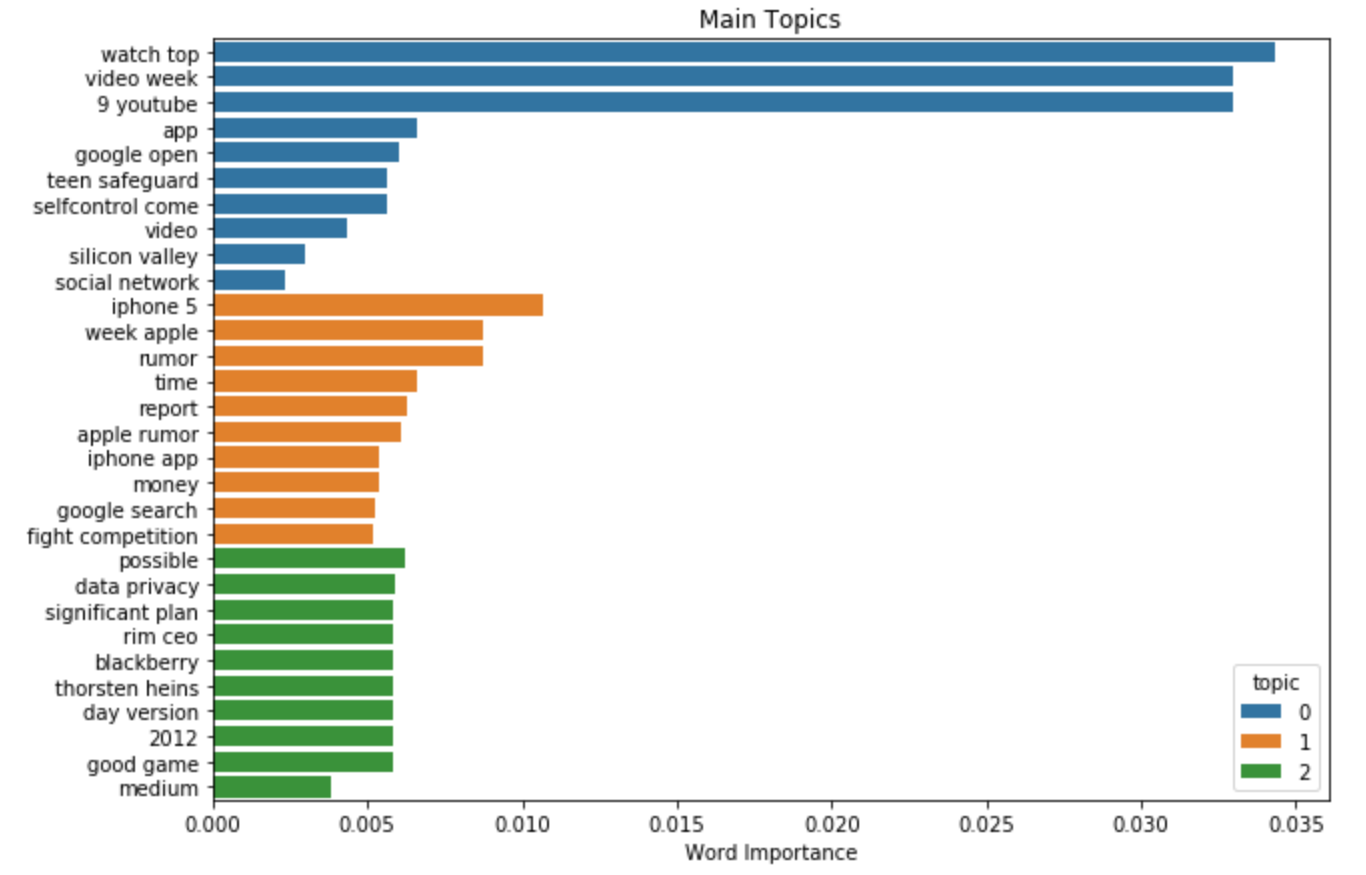### 结论

http://panchuang.net/

sklearn机器学习中文官方文档：
http://sklearn123.com/

http://docs.panchuang.net/

posted @ 2020-07-02 15:50  人工智能遇见磐创  阅读(393)  评论(0编辑  收藏